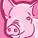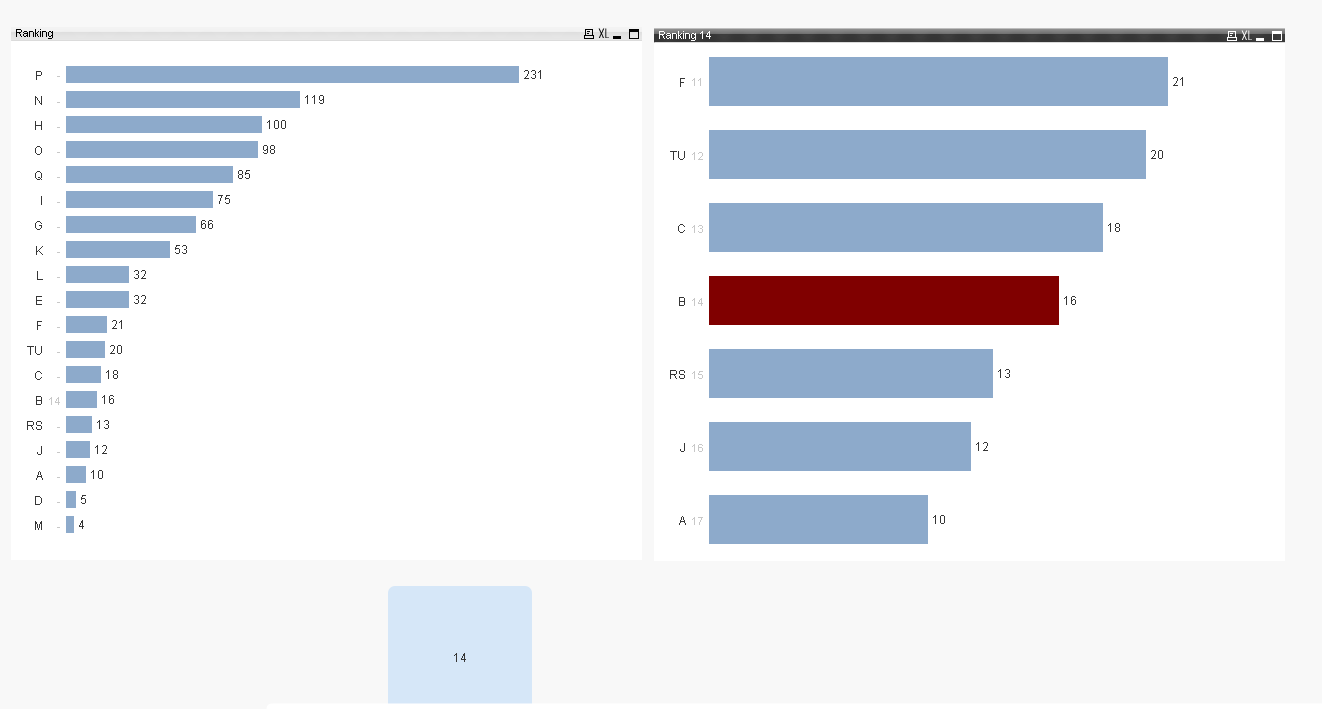# QlikView App Dev

Discussion Board for collaboration related to QlikView App Development.

Announcements
Action-Packed Learning Awaits! QlikWorld 2023. April 17 - 20 in Las Vegas: REGISTER NOW
cancel
Showing results for
Did you mean:Contributor II

## Subset n values above and below rank selection

Hi community,

After selecting a project, I will like to filter my projects and show only the n+[Selections Rank]  and n-[Selections Rank] values.

Example:

if my selection has rank position  10, I will expect a bar chart to show projects with rank 5 6 7 8 9 10 11 12 13 14 15. (Keeping the original total ranks).

Here is part  of the expression I tried:

=if(aggr( rank(sum({1} Cost), 1),Project) <=

aggr( rank(total sum(  {1<Project  = Project> } Cost)), Project),

Project)

Information:

[

Project, Date, Cost

A, 01.01.2018, 10

B, 02.01.2018, 16

C, 03.01.2018, 18

D, 04.01.2018, 5

E, 05.01.2018, 32

F, 29.01.2018, 21

G, 01.02.2018, 66

H, 02.02.2018, 100

I, 03.02.2018, 75

J, 04.02.2018, 12

K, 26.02.2018, 53

L, 27.03.2018, 32

M, 28.03.2018, 4

N, 01.03.2018, 6

N, 02.03.2018, 74

N, 03.03.2018, 39

O, 04.03.2018, 98

P, 28.03.2018, 84

P, 29.03.2018, 92

P, 01.04.2018, 55

Q, 02.04.2018, 44

Q, 03.04.2018, 41

RS, 04.04.2018, 13

TU, 05.04.2018, 20

];

UPDATE:

If I store the rank of my selection on a variable the projects get filtered but the chart doesnt show the rank for the other projects.

=if(aggr( rank(sum({1} Cost), 1),Project) >= vTest-5 and aggr( rank(sum({1} Cost), 1),Project) <= vTest+5,

Project)

1 Solution

Accepted SolutionsMVP

Try this

Only({1} Aggr(Rank(TOTAL Sum({<Project>} Cost), 1, Project), Project))

or this

Only({<Project>} Aggr(Rank(TOTAL Sum({<Project>} Cost), 1, Project), Project))3 RepliesMVP

Try this

Only({1} Aggr(Rank(TOTAL Sum({<Project>} Cost), 1, Project), Project))

or this

Only({<Project>} Aggr(Rank(TOTAL Sum({<Project>} Cost), 1, Project), Project))Contributor II
Author

Hi Sunny,

it looks bettter now. Can you explain the effect of only in the expression?

Do you have any idea why without a variable the dimension doesn´t work?

Thanks !MVP

If you don't want to use variable, then you can try this

=If(Aggr(Rank(Sum({1} Cost), 1), Project) >= Only(TOTAL Aggr(Rank(TOTAL Sum({1<Project>} Cost)), Project))-3 and Aggr(Rank(Sum({1} Cost), 1), Project) <= Only(TOTAL Aggr(Rank(TOTAL Sum({1<Project>} Cost)), Project))+3, Project)

 Can you explain the effect of only in the expression?

It was needed because I needed to use set analysis to avoid selection in Project for the aggregation outside of Aggr(). When you don't have any aggregation, it is equivalent of having Only()... so what I mean is that these two are the same exact thing

Only(Aggr(Rank(TOTAL Sum({<Project>} Cost), 1, Project), Project))

and

Aggr(Rank(TOTAL Sum({<Project>} Cost), 1, Project), Project)

But, in order to avoid selection in Project for the outer aggregation, I used Only with {1} or {<Project>}

Does that make sense?Community Browser